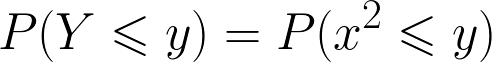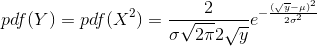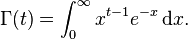let X with pdf of f(x) be

f(x) = \frac{1}{{\sigma \sqrt {2\pi }} }e^{ – \frac  {{(x – \mu)}^2 }{2\sigma^2} }Y=X^2

dy/dx=2x

dx=dy/2x

Cumulative probability function of  of Y will

beBecause of symmetry of X and X^2=P(-\sqrt{y}\leqslant x \leqslant \sqrt{y})Y=X^2

dy/dx=2x

dx=dy/2x

G(y)=2\int_{0}^{\sqrt{y}}{\frac{1}{{\sigma \sqrt {2\pi }} }e^{ – \frac  {{(x – \mu)}^2 }{2\sigma^2} }}dxtherefore

pdf for ypdf(y)=\frac{2}{\sigma \sqrt {2\pi } 2 \sqrt y} {e^{ – \frac  {{(\sqrt y – \mu)}^2 }{2\sigma^2} }}pdf(y)=\frac{2}{ 2\sqrt {y}\sqrt {2\pi} } {e^{ – \frac  {y }{2} }}

Below we can show that the formula above a Chi squared distribution.

For Z=(x-mu/Sigma)

=======================================================

if mu=0 and sigma=1 which means standard normal distribution  Y=z^2

we can replace Sqrt(pi) with gamma functionwe knowthenpdf(Z)=\frac{y^{(\frac {k}{2}-1)}{e^{ – \frac  {z }{2} }}}{ 2^{(1/2)} \Gamma (1/2) }

compared with general Gamma distributionThis is Gamma distribution shape alpha  1/2 and scale  beta of 2

mean of 1 and variance of 2

and we call it Chi square distribution with 1 degree of freedom.